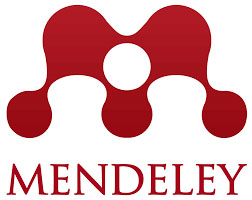rscosan.com

# Paper: Factorization method for difference equations of hypergeometric type on nonuniform lattices

 IOP PUBLISHING LTD (Bristol, UK) | ISSN: 0305-4470 | JCR® 2001 Impact Factor: 1.453 - PHYSICS, MATHEMATICAL — position: 7/29 (Q1/T1) Factorization method for difference equations of hypergeometric type on nonuniform lattices## Factorization method for difference equations of hypergeometric type on nonuniform lattices

Álvarez-Nodarse, Renato and Costas-Santos, Roberto S. Journal of Physics A: Mathematical and General volume 34, no. 27 (2001), 5551 — 5569

## Abstract

We study the factorization of the hypergeometric-type difference equation of Nikiforov and Uvarov on nonuniform lattices. An explicit form of the raising and lowering operators is derived and some relevant examples are given.

218 KB Preprint (PDF, 21 pages)

## BibTeX

```@article {MR1856663,
AUTHOR={\'{A}lvarez-Nodarse, R. and Costas-Santos, R. S.},
TITLE={Factorization method for difference equations of hypergeometric type on nonuniform lattices},
JOURNAL={J. Phys. A},
FJOURNAL={Journal of Physics A. Mathematical and General},
VOLUME={34},
YEAR={2001},
NUMBER={27},
PAGES={5551--5569},
ISSN={0305-4470},
MRCLASS={39A13 (33C45 33D45)},
MRNUMBER={1856663},
ZBL={0994.39001},
MRREVIEWER={A. M. Gavrilik},
DOI={10.1088/0305-4470/34/27/306},
URL={https://doi.org/10.1088/0305-4470/34/27/306},
}
```